## 5.285. nset_of_consecutive_values

Origin

N. Beldiceanu

Constraint

$\mathrm{𝚗𝚜𝚎𝚝}_\mathrm{𝚘𝚏}_\mathrm{𝚌𝚘𝚗𝚜𝚎𝚌𝚞𝚝𝚒𝚟𝚎}_\mathrm{𝚟𝚊𝚕𝚞𝚎𝚜}\left(𝙽,\mathrm{𝚅𝙰𝚁𝙸𝙰𝙱𝙻𝙴𝚂}\right)$

Arguments
 $𝙽$ $\mathrm{𝚍𝚟𝚊𝚛}$ $\mathrm{𝚅𝙰𝚁𝙸𝙰𝙱𝙻𝙴𝚂}$ $\mathrm{𝚌𝚘𝚕𝚕𝚎𝚌𝚝𝚒𝚘𝚗}\left(\mathrm{𝚟𝚊𝚛}-\mathrm{𝚍𝚟𝚊𝚛}\right)$
Restrictions
 $𝙽\ge 1$ $𝙽\le |\mathrm{𝚅𝙰𝚁𝙸𝙰𝙱𝙻𝙴𝚂}|$ $\mathrm{𝚛𝚎𝚚𝚞𝚒𝚛𝚎𝚍}$$\left(\mathrm{𝚅𝙰𝚁𝙸𝙰𝙱𝙻𝙴𝚂},\mathrm{𝚟𝚊𝚛}\right)$
Purpose

$𝙽$ is the number of set of consecutive values used by the variables of the collection $\mathrm{𝚅𝙰𝚁𝙸𝙰𝙱𝙻𝙴𝚂}$.

Example
 $\left(2,〈3,1,7,1,1,2,8〉\right)$ $\left(7,〈3,1,5,7,9,11,13〉\right)$ $\left(1,〈3,3,3,3,3,3,3〉\right)$

In the first example, the two parts $3,1,1,1,2$ and $7,8$ take respectively their values in the following sets of consecutive values $\left\{1,2,3\right\}$ and $\left\{7,8\right\}$. Consequently, the corresponding $\mathrm{𝚗𝚜𝚎𝚝}_\mathrm{𝚘𝚏}_\mathrm{𝚌𝚘𝚗𝚜𝚎𝚌𝚞𝚝𝚒𝚟𝚎}_\mathrm{𝚟𝚊𝚕𝚞𝚎𝚜}$ constraint holds since its first argument $𝙽=2$ is set to the number of sets of consecutive values.

Typical
 $𝙽>1$ $|\mathrm{𝚅𝙰𝚁𝙸𝙰𝙱𝙻𝙴𝚂}|>1$ $\mathrm{𝚛𝚊𝚗𝚐𝚎}$$\left(\mathrm{𝚅𝙰𝚁𝙸𝙰𝙱𝙻𝙴𝚂}.\mathrm{𝚟𝚊𝚛}\right)>1$
Symmetries
• Items of $\mathrm{𝚅𝙰𝚁𝙸𝙰𝙱𝙻𝙴𝚂}$ are permutable.

• All occurrences of two distinct values of $\mathrm{𝚅𝙰𝚁𝙸𝙰𝙱𝙻𝙴𝚂}.\mathrm{𝚟𝚊𝚛}$ can be swapped.

• One and the same constant can be added to the $\mathrm{𝚟𝚊𝚛}$ attribute of all items of $\mathrm{𝚅𝙰𝚁𝙸𝙰𝙱𝙻𝙴𝚂}$.

Arg. properties

Functional dependency: $𝙽$ determined by $\mathrm{𝚅𝙰𝚁𝙸𝙰𝙱𝙻𝙴𝚂}$.

Usage

Used for specifying the fact that the values have to be used in a compact way is achieved by setting $𝙽$ to 1.

Counting
 Length ($n$) 2 3 4 5 6 7 8 Solutions 9 64 625 7776 117649 2097152 43046721

Number of solutions for $\mathrm{𝚗𝚜𝚎𝚝}_\mathrm{𝚘𝚏}_\mathrm{𝚌𝚘𝚗𝚜𝚎𝚌𝚞𝚝𝚒𝚟𝚎}_\mathrm{𝚟𝚊𝚕𝚞𝚎𝚜}$: domains $0..n$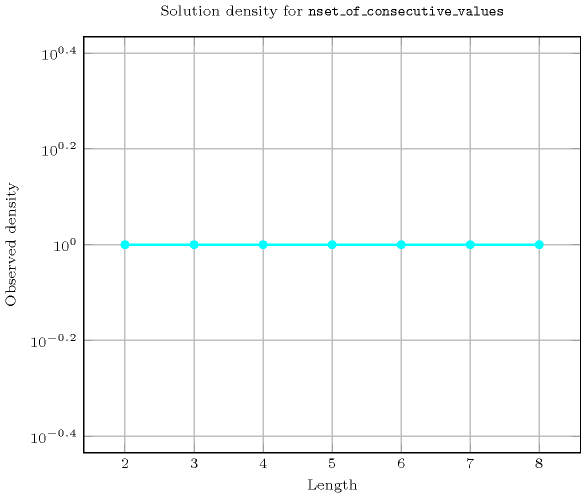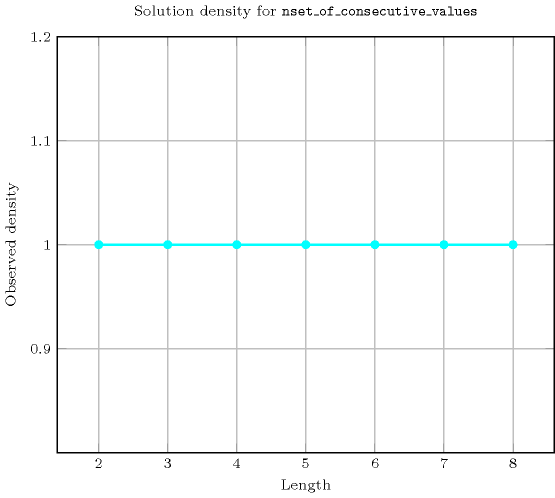Length ($n$)2345678
Total9646257776117649209715243046721
 Parameter value

17342171716161591763662187637
223037247406501096906615695624
3--3613203492084252019989900
4----15601092005047560
5------126000

Solution count for $\mathrm{𝚗𝚜𝚎𝚝}_\mathrm{𝚘𝚏}_\mathrm{𝚌𝚘𝚗𝚜𝚎𝚌𝚞𝚝𝚒𝚟𝚎}_\mathrm{𝚟𝚊𝚕𝚞𝚎𝚜}$: domains $0..n$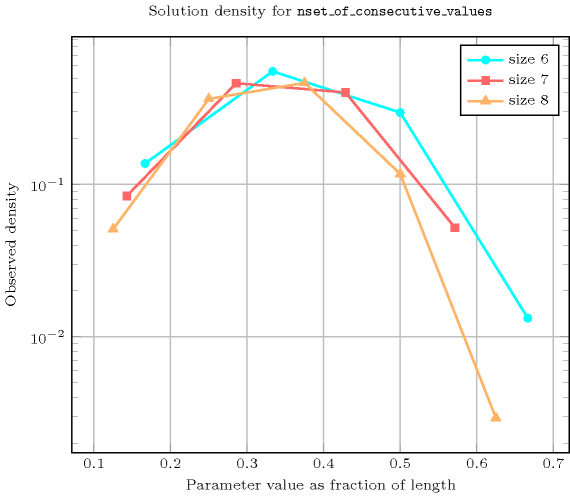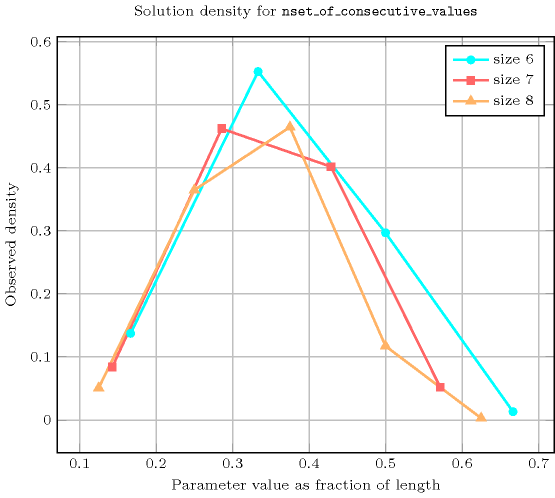Keywords
Arc input(s)

$\mathrm{𝚅𝙰𝚁𝙸𝙰𝙱𝙻𝙴𝚂}$

Arc generator
$\mathrm{𝐶𝐿𝐼𝑄𝑈𝐸}$$↦\mathrm{𝚌𝚘𝚕𝚕𝚎𝚌𝚝𝚒𝚘𝚗}\left(\mathrm{𝚟𝚊𝚛𝚒𝚊𝚋𝚕𝚎𝚜}\mathtt{1},\mathrm{𝚟𝚊𝚛𝚒𝚊𝚋𝚕𝚎𝚜}\mathtt{2}\right)$

Arc arity
Arc constraint(s)
$\mathrm{𝚊𝚋𝚜}\left(\mathrm{𝚟𝚊𝚛𝚒𝚊𝚋𝚕𝚎𝚜}\mathtt{1}.\mathrm{𝚟𝚊𝚛}-\mathrm{𝚟𝚊𝚛𝚒𝚊𝚋𝚕𝚎𝚜}\mathtt{2}.\mathrm{𝚟𝚊𝚛}\right)\le 1$
Graph property(ies)
$\mathrm{𝐍𝐒𝐂𝐂}$$=𝙽$

Graph model

Since the arc constraint is symmetric each strongly connected component of the final graph corresponds exactly to one connected component of the final graph.

Parts (A) and (B) of Figure 5.285.1 respectively show the initial and final graph associated with the first example of the Example slot. Since we use the $\mathrm{𝐍𝐒𝐂𝐂}$ graph property, we show the two strongly connected components of the final graph.

##### Figure 5.285.1. Initial and final graph of the $\mathrm{𝚗𝚜𝚎𝚝}_\mathrm{𝚘𝚏}_\mathrm{𝚌𝚘𝚗𝚜𝚎𝚌𝚞𝚝𝚒𝚟𝚎}_\mathrm{𝚟𝚊𝚕𝚞𝚎𝚜}$ constraint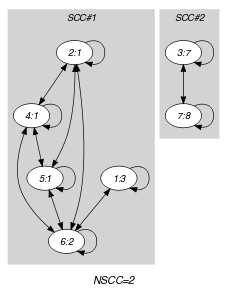(a) (b)